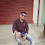## Yii2: Validate value without model (Ad Hoc Validation)

Sometimes you need to do ad hoc validation for values that are not bound to any model.
If you only need to perform one type of validation (e.g. validating email addresses), you may call the [[yii\validators\Validator::validate()|validate()]] method of the desired validator, like the following:
```\$email = 'test@example.com';
\$validator = new yii\validators\EmailValidator();

if (\$validator->validate(\$email, \$error)) {
echo 'Email is valid.';
} else {
echo \$error;
}```
Note: Not all validators support this type of validation. An example is the unique core validator which is designed to work with a model only.
If you need to perform multiple validations against several values, you can use [[yii\base\DynamicModel]] which supports declaring both attributes and rules on the fly. Its usage is like the following:
```public function actionSearch(\$name, \$email)
{
\$model = DynamicModel::validateData(compact('name', 'email'), [
[['name', 'email'], 'string', 'max' => 128],
['email', 'email'],
]);

if (\$model->hasErrors()) {
// validation fails
} else {
// validation succeeds
}
}```
The [[yii\base\DynamicModel::validateData()]] method creates an instance of `DynamicModel`, defines the attributes using the given data (`name` and `email` in this example), and then calls [[yii\base\Model::validate()]] with the given rules.
Alternatively, you may use the following more "classic" syntax to perform ad hoc data validation:
```public function actionSearch(\$name, \$email)
{
\$model = new DynamicModel(compact('name', 'email'));
\$model->addRule(['name', 'email'], 'string', ['max' => 128])
->validate();

if (\$model->hasErrors()) {
// validation fails
} else {
// validation succeeds
}
}```
After validation, you can check if the validation succeeded or not by calling the [[yii\base\DynamicModel::hasErrors()|hasErrors()]] method, and then get the validation errors from the [[yii\base\DynamicModel::errors|errors]] property, like you do with a normal model. You may also access the dynamic attributes defined through the model instance, e.g., `\$model->name` and `\$model->email`.
1.